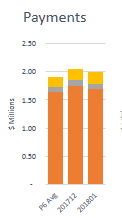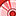Announcements
You can succeed best and quickest by helping others to succeed. Join the conversation.
cancel
Showing results for
Did you mean:Contributor III

## Implementing Bar Chart with Uniquely Grouped Dimensions

Hello,

I am attempting to recreate this bar chart that was originally made in excel. The chart has 3 different expressions for each column showing a 6 month avg, a summed total for previous month, and a summed total for current month. The chart is stacked by the financial class (denoted by orange, grey, and yellow).I was able to get this far with the below three expressions but I am not sure how to go about implementing the dimensions so that it shows the same type of stacked bar chart above. When I add my desired dimension to the chart, I either get each expression for each value in the dimension, or I get the 3 expressions stacked for each dimension value.Expression 1 (blue): =num(sum({<_M={6,5,4,3,2,1}>} [Payments])/6,'\$#,##0')

Expression 2 (red): =sum({<_M={6}>} [Payments])

Expression 3 (green): =sum({<_M={7}>} [Payments])

Any help towards recreating the first chart would be greatly appreciated!

1 Solution

Accepted SolutionsSpecialist III

I created a dimension called period, you would want to use members from your calendar.

Per:

Per

'PG Avg'

'201712'

'201801'

];

Data:

FinClass,Mth,Amt

1,6,100

1,5,100

1,4,100

1,3,100

1,2,100

1,1,105

1,7,100

2,7,50

2,6,50

2,5,50

2,4,50

2,3,50

2,2,50

2,1,50

3,7,25

3,6,25,

3,5,25

];

The chart expressions uses an IF statement:

=If(Per = 'PG Avg',Sum({<Mth={'6','5','4','3','2','1'}>} Amt)/6,If(Per='201712',Sum({<Mth={'6'}>} Amt),Sum({<Mth={'7'}>} Amt)))

The Background Color definition:

=If(FinClass = 1,Red(),If(FinClass = 2,DarkGray(),Yellow()))2 RepliesSpecialist III

I created a dimension called period, you would want to use members from your calendar.

Per:

Per

'PG Avg'

'201712'

'201801'

];

Data:

FinClass,Mth,Amt

1,6,100

1,5,100

1,4,100

1,3,100

1,2,100

1,1,105

1,7,100

2,7,50

2,6,50

2,5,50

2,4,50

2,3,50

2,2,50

2,1,50

3,7,25

3,6,25,

3,5,25

];

The chart expressions uses an IF statement:

=If(Per = 'PG Avg',Sum({<Mth={'6','5','4','3','2','1'}>} Amt)/6,If(Per='201712',Sum({<Mth={'6'}>} Amt),Sum({<Mth={'7'}>} Amt)))

The Background Color definition:

=If(FinClass = 1,Red(),If(FinClass = 2,DarkGray(),Yellow()))Contributor III
Author

Perfect, thank you!Community Browser